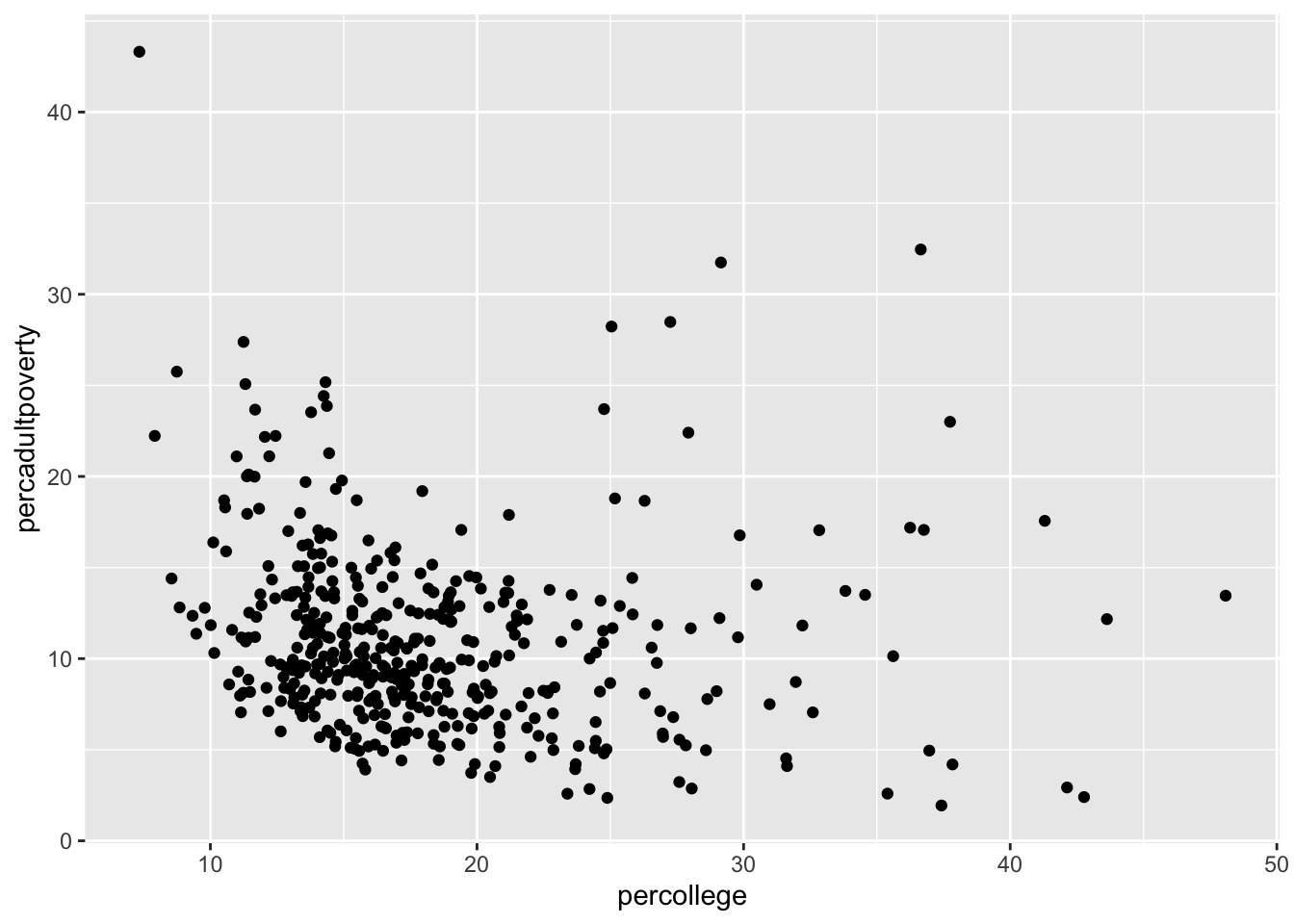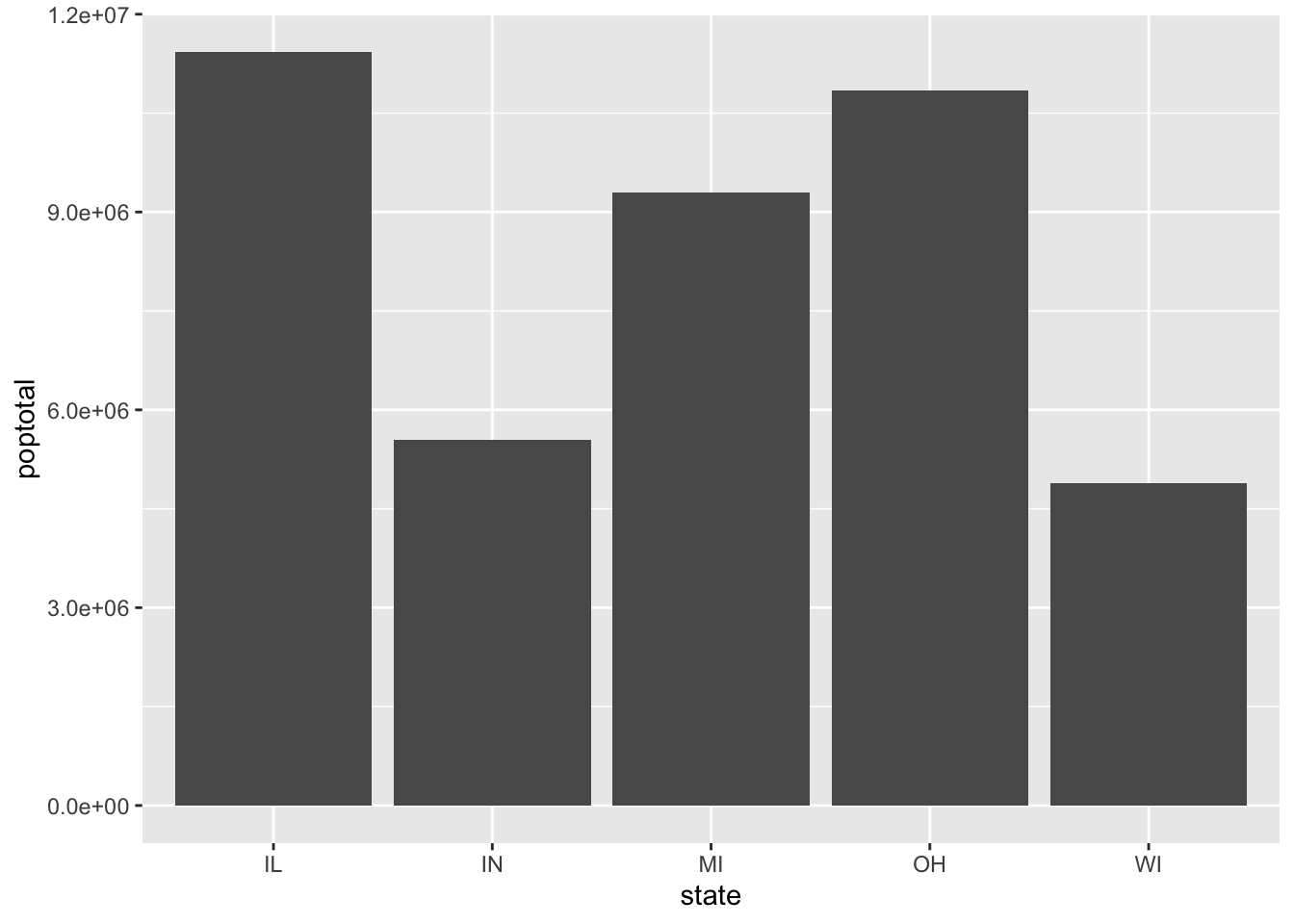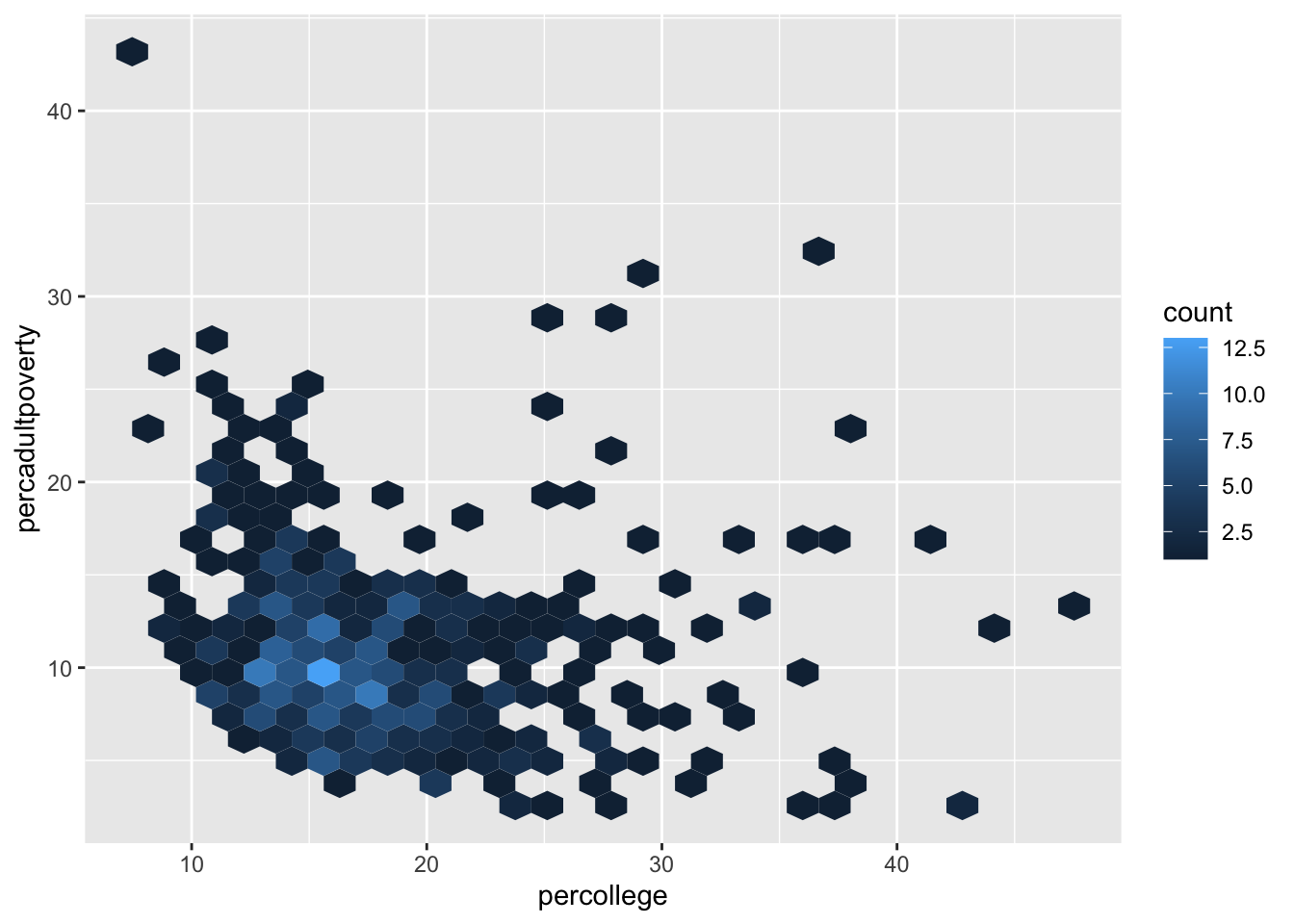``library(tidyverse)``

# A GRAMMAR OF GRAPHICS

(Hadley Wickham rev., 2010) to describe the components of a plot:

• The data being plotted

• The geometric objects (e.g., circles, lines) that appear on the plot

• The aesthetics (appearance) of the geometric objects, and the mappings from variables in the data to those aesthetics

• A position adjustment for placing elements on the plot so they don’t overlap

• A scale (e.g., a range of values) for each aesthetic mapping used

• A coordinate system used to organize the geometric objects

• The facets or groups of data shown in different plots

ggplot2 further organizes these components into layers, where each layer displays a single type of (highly configurable) geometric object.

(data,aesthetics) + geometry

``````ggplot(data = <DATA>) +
<GEOM_FUNCTION>(mapping = aes(<MAPPINGS>))``````
• 先用 `ggplot()` 繪製一個圖布 canvas（吃一個 dataframe 引數），建立一個座標系統，讓你之後可以疊加不同的圖層上去。

• `ggplot2` 函式庫提供許多 `geom` 函式， 每個皆可以為圖表新增不同類型的圖層。

• 每個 `geom` 函式都接受一個 `mapping` 引數，定義了資料集中的變數是如何被映射 (mapped) 到視覺特性上。
``````#?midwest (ggplot2 built-in data)
#The data set contains information on each of 437 counties in 5 states in the midwestern United States (specifically, Illinois, Indiana, Michigan, Ohio, and Wisconsin)

# Plot the `midwest` data set, with the percentag of people with a college education on the x-axis and
# percentage of adult poverty on the y-axis
ggplot(data = midwest) +
geom_point(mapping = aes(x = percollege, y = percadultpoverty)) # ``````1. The `ggplot()` function is passed the data frame to plot as the named data argument (it can also be passed as the first positional argument). Calling this function creates the blank canvas on which the visualization will be created.

2. You specify the type of geometric object (sometimes referred to as a `geom`) to draw by calling one of the many geom_ functions — in this case, `geom_point()`. Functions to render a layer of geometric objects all share a common prefix (geom_), followed by the name of the kind of geometry you wish to create. For example, `geom_point()` will create a layer with “point” (dot) elements as the geometry.

3. In each `geom_ function`, you must specify the aesthetic mappings, which specify how data from the data frame will be mapped to the visual aspects of the geometry. These mappings are defined using the `aes()` (aesthetic) function.

• The `aes()` function takes a set of named arguments (like a list), where the argument name is the visual property to map to, and the argument value is the data feature (i.e., the column in the data frame) to map from. The value returned by the `aes()` function is passed to the named mapping argument (or passed as the first positional argument).
1. add layers of geometric objects to the plot by using the addition (+) operator.

## 幾何物件設定 specifying geometrics

using the appropriate `geom_` function including:

• `geom_point()` for drawing individual points 點 (e.g., for a scatterplot)

• `geom_line()` for drawing lines 線 (e.g., for a line chart)

• `geom_smooth()` for drawing smoothed lines 平滑線 (e.g., for simple trends or approximations)

• `geom_col()` for drawing columns 行(e.g., for a bar chart)

• `geom_polygon()` for drawing arbitrary shapes 多邊形 (e.g., for drawing an area in a coordinate plane)

Each of these `geom_` functions requires as an argument a set of aesthetic mappings (defined using the `aes()` function.

Since graphics are two-dimensional representations of data, almost all geom_ functions require an x and y mapping.

``````# A bar chart of the total population of each state
# The `state` is mapped to the x-axis, and the `poptotal` is mapped
# to the y-axis

ggplot(data = midwest) +
geom_col(mapping = aes(x = state, y = poptotal))````````````# A hexagonal aggregation that counts the co-occurrence of college
# education rate and percentage of adult poverty
# 六角形
ggplot(data = midwest) +
geom_hex(mapping = aes(x = percollege, y = percadultpoverty))``````• What makes this really powerful is that you can add multiple geometries to a plot. This allows you to create complex graphics showing multiple aspects of your data
``````# A plot with both points and a smoothed line
ggplot(data = midwest) +
geom_point(mapping = aes(x = percollege, y = percadultpoverty)) +
geom_smooth(mapping = aes(x = percollege, y = percadultpoverty))``````
``## `geom_smooth()` using method = 'loess' and formula 'y ~ x'``